# RBSE Solutions For Class 12 Physics Chapter 3: Electric Potential | Textbook Important Questions & Answers

The answers are provided for all the questions of Chapter 3 Physics of RBSE Class 12. Students can go through these questions to understand the concepts better and score well in the board examination and entrance examinations for various professional courses. These solutions are provided by the team of experts to provide the best and accurate solutions to the questions. Do check BYJU’S RBSE Class 12 solutions page to get the answers for textbook questions of all subjects.

## Multiple Choice Questions

Q1: At a certain distance from a point charge electric field and potential are 50 V and 300 V respectively, this distance is

1. 9m
2. 15m
3. 6m
4. 3m

Q2: Four charges are placed on corners of a square as shown in the figure. Let the electric field and potential at its centre are E and V. If the charges at A and B are interchanged with charges placed at C and D

Then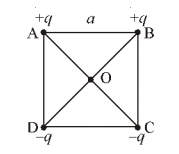1. E remains the same but V is changed
2. Both E and V are changed
3. Both E and V are unchanged
4. E is changed but V remains the same

Q3: The electric potential at some point in an electric field is 200 v. The work done in moving an electron from infinity to that point is

1. -3.2 x 10-17J
2. 200 J
3. -200J
4. 100 J

Q4: The electric potential at the surface of a charged spherical hollow conductor of radius 2m is 500 V. The potential at a distance 1.5 m from the centre is

1. 375 V
2. 250 V
3. Zero
4. 500 V

Q5: An ∝ particle is moved from rest from a point where potential is 70 V to another point having potential 50 V. The kinetic energy of ∝ particle at the second point is

1. 20 eV
2. 40 eV
3. 20 MeV
4. 40 MeV

Q6: In the region where the electric field intensity E is zero, the electric potential varies with distance according to

1. V ∝ 1/r
2. V ∝ 1/r2
3. V = Zero
4. V = constant

Q7: The electric potential function for some electric field is defined y V =-5x +3y +z. The intensity of the electric field (in S.I.units) at point (x,y,z) is

1. 3
2. 4
3. 5
4. 7

Q1: Mention whether the electric potential is a scalar or vector quantity

Answer: The electric potential is a scalar quantity

Q2: Give the definition of electric potential

Answer: Electric potential is the amount of work done by an external agent to bring a unit positive charge from a reference point to a specified point.

Q3: Can two equipotential surfaces intersect each other at some point?

Answer: Two equipotential surfaces cannot intersect each other at the same point. Otherwise, there will be two values for the electric field.

Q4: What will be the electric potential due to a charge, at infinity

Answer: The electric potential at infinity is zero

Q5: Can electric potential at some point in free space be zero though the electric field may not be zero at that point? Give an example

Answer: Yes, electric potential can be zero along the equatorial line of an electric dipole but the electric field is not zero

Q6: Can the electric field at some point be zero though the electric potential is not zero? Give an example.

Answer: There are 2 examples of an electric field becoming zero when an electric potential is not zero

1. The value of the electric field is zero at the midpoint of the line joining two charges but the electric potential is not zero
2. The electric field is zero in a spherical shell but the electric potential is not zero

Q7: What will be the work done in moving 200 μC charges in moving it from one point to another point at a distance of 10 cm on the same equipotential surface?

Answer: The work done will be zero since the potential difference between any two points on a given equipotential surface is zero.

ΔV = 0

W = qΔV =0

Q8: What is the shape of the equipotential surface for the following

1. Due to a point charge
2. For a uniform electric field

(a) Spherical surface centred at the point charge

(b) Parallel planes oriented normal to the electric field

Q9: What is the potential energy of an electric dipole placed parallel to an electric field?

Answer: The electric potential is aligned along the direction of the field. Therefore, potential energy is given by U= -p.E.

where p is the electric dipole moment, E is the electric field

Q10: The electric potential at the surface of a charged spherical conductor of radius

10 cm is 15 V. What is the electric potential at its centre.

Q1: What is meant by the equipotential surface. Draw equipotential surfaces for a point charge.

Answer: The equipotential surface has a constant potential and the potential difference between any two points on the surface is zero.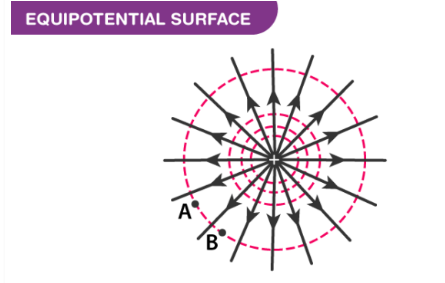Q2: Determine the expression for potential energy for a system of three-point charges

Answer: Consider the charges kept at three vertices of a triangle as shown in the figure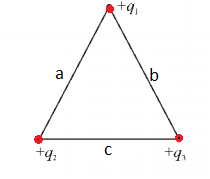Let the q1 be the charge present at one of the vertices of the triangle. The amount of work done in bringing q2 is given by W1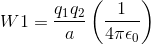The work done in bringing the charge q3 at the third vertex of the triangle is given by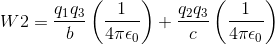The total work done is given by

W =W1+W2

The total work done is also equal to the total potential (U)

Therefore,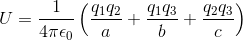Q3: The electric potential throughout the volume of a charged conductor is the same as that on its surface, why?

Answer: The Electric field inside a conductor is zero so the work done will be zero. Therefore, the change in potential energy ΔU is also zero. Suppose U1 is the potential at a point inside the conductor and U2 is the potential on the surface, then U2 – U1 =0 or U2 = U1. This means that electric potential throughout the volume of a charged conductor is the same as that on its surface.

Q4: Derive the relation between electric potential and field

Answer: Let us consider two points A and B separated by the very small distance d. Since the distance between the points is small the electric field is considered uniform along AB.

The work done in moving a unit positive charge from A to B against the electric field is

W = – Edx

The negative sign is to show that the work is done against the electric field.

The work done is equal to the potential difference between the two points

Therefore,

ΔU = – Edx

Rearranging the above equation we have

E = – ΔU/dx

“Electric field is the negative space derivative of electric potential.”

Q5: Derive the expression for work done in rotating an electric dipole in a uniform electric field.

Torque is the force that causes a dipole to rotate about an axis. The torque to rotate a dipole is given by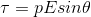Where p is the dipole moment, E is the electric field and θ is the angle made by the dipole with the electric field.

The work done in rotating the dipole through a small angle dθ is given by

dW = τdθ

So the work done in rotating from the angular position θ = 0 to θ = θ0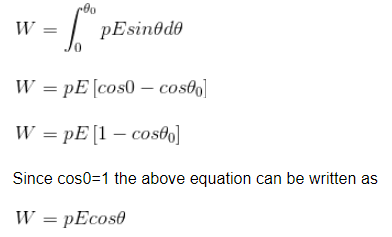Q6: Show that no work is done in moving a test charge from one point to another on the equipotential surface.

Answer: Equipotential meaning the potential is constant all over the surface. There will not be any potential difference when a test charge is moved from one point to another. When there is no potential difference then the work done to move the charge is also zero.

Work done W = qΔU

The potential difference on an equipotential surface ΔU=0

Therefore W=0

Q7: Write two properties of an equipotential surface

• Two equipotential surfaces can never intersect.
• The electric field is always perpendicular to an equipotential surface.

## Essay Type Questions

Q1: Derive an expression for electric potential at a point due to an electric dipole. Show that potential is maximum for axial point while is zero for an equatorial line.

Answer: Let us consider two charges -q and +q at points A and B at a distance 2a. Let us consider a point P at a distance r from the centre of the dipole. The line OP makes an angle θ with the axis of the dipole.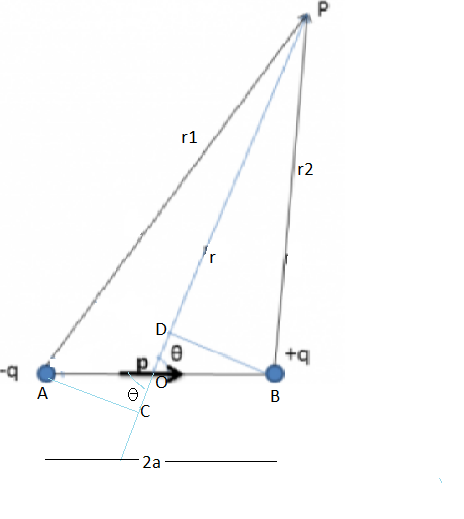The potential at P due to the charge -q at A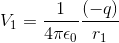The potential at P due to charge +q at B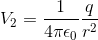The potential at P due to the dipole is given by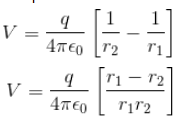To determine the value of r1 – r2 and r1r2 let us draw a perpendicular line BD and AC.

Therefore,

OP = OD+DP

r= acosθ + r2

Since DP ≈ BP ≈ r2

From the triangle BOD, OD= acosθ

Therefore r2 = r- acosθ

CP = OP+OC

r1 =r + acosθ

Since AP≈CP

From the triangle AOC, OC= acosθ

Therefore r1 – r2 =2acosθ

And r1r2 =r2

Substituting these values we get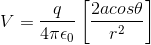Since p = 2aq we get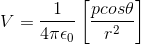For the axial point θ = 0,Therefore Cos 0=1

Hence, the equation becomes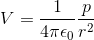For the equatorial points θ = 90 degrees, therefore cos 90=0

Hence V = 0

Q2: Give the expressions for electric potential due to a charged spherical shell at points (i) outside (ii) on the surface (iii) inside the shell.

Let us consider a spherical shell of radius R. The charges Q equally distributed on the surface of the shell.

(i) Point outside the shell

For a point outside the shell, the charged shell acts as a point charge. Let us consider a point at a distance r from the centre of the sphere.

The potential energy at this point is given by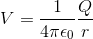(ii) Point on the surface of the shell

The radius of the sphere is given as R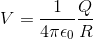(iii) Point inside the sphere

In the region inside the sphere, there are zero electric fields and the electric potential is constant. Therefore the electric field is the same as that of the surface

Therefore, the value is given by